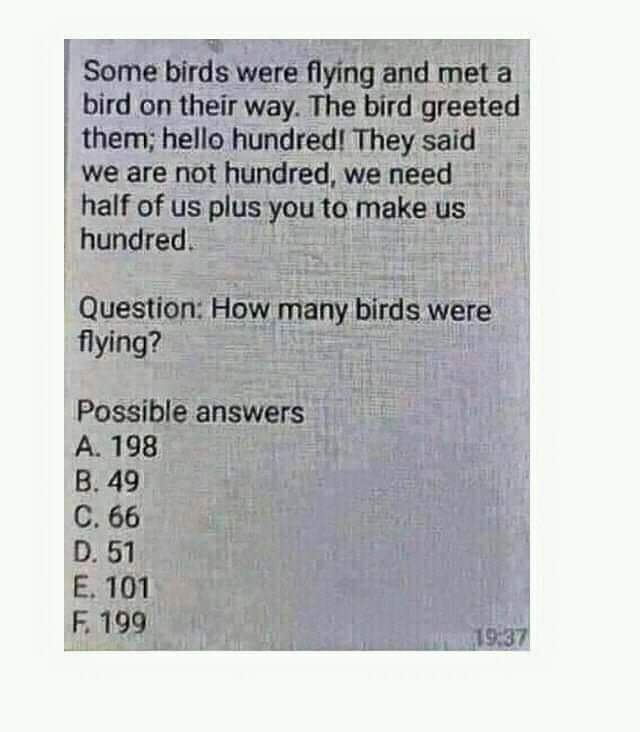# Birds Application

• MHB
• mathland

#### mathlandLet b = birds

Met a bird = (b + 1)

Half of us plus you = (b + 1)/2 + (b + 1)

The equation is:

(b + 1)/2 + (b + 1) = 100

Yes?

View attachment 10948

Let b = birds

Met a bird = (b + 1)

Half of us plus you = (b + 1)/2 + (b + 1)

The equation is:

(b + 1)/2 + (b + 1) = 100

Yes?
No! the flock of birds is talking to the one new bird. When it says "us" it means the original flock, not with the new bird. "Half of us" is b/2. "Half of us plus you" is b/2+ 1.

Your equation is b/2+ 1= 100.

No! the flock of birds is talking to the one new bird. When it says "us" it means the original flock, not with the new bird. "Half of us" is b/2. "Half of us plus you" is b/2+ 1.

Your equation is b/2+ 1= 100.

Another great math skill is the ability to create an equation from the given information in applications.